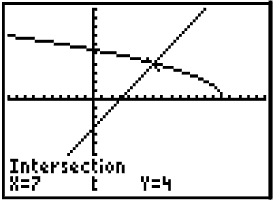Example:  Solve the following equation algebraically and check.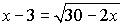Algebraic Solution: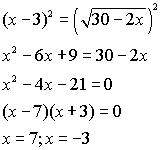Graphical check: Enter the left side of the equation into Y1. Enter the right side of the equation into Y2. Use the INTERSECT option (2nd Trace #5).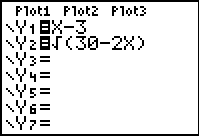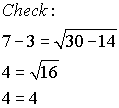extraneous root The calculator clearly shows that there is only one solution (x = 7), thus showing that x = -3 is an extraneous root.